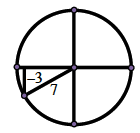### Home > PC > Chapter 6 > Lesson 6.3.2 > Problem6-130

6-130.

If $\sin x = −\frac { 3 } { 7 }$, $x ∈ [π, \frac { 3 \pi } { 2 }]$, find exact values for $\sin 2x$ and $\cos 2x$.

Draw and label a circle with the reference angle in the correct quadrant.
Find the length of the unlabeled side.

Use the Double Angle Formula for the sine to find $\sin\left(2x\right)$.Use one of the Double Angle Formulas for the cosine to find $\cos\left(2x\right)$.

Double - Angle Formulas: Sine
$\sin \left(2α\right) = 2 \sin\left(α\right)\cos \left(α\right)$

Double - Angle Formulas: Cosine
1. $\cos \left(2α\right) = 1 − 2 \sin^{2}\left(α\right)$
2. $\cos 2\left(α\right) = 2\cos^{2}\left(α\right) − 1$
3. $\cos \left(2α\right) = \cos^{2}\left(α\right) − \sin^{2}\left(α\right)$